# High School Math : Understanding Secant, Cosecant, and Cotangent

## Example Questions

### Example Question #1 : Understanding Secant, Cosecant, And Cotangent

An angle has a cosine of. What will its cosecant be?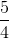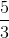Explanation:

The problem tells us that the cosine of the angle will be. Cosine is the adjacent over the hypotenuse. From here we can use the Pythaogrean theorem: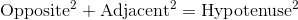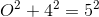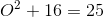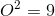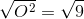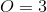Now we know our opposite, adjacent, and hypotenuse.

The cosecant is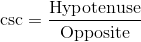.

From here we can plug in our given values.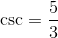### Example Question #2 : Understanding Secant, Cosecant, And Cotangent

Which of these is equal to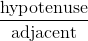for angle?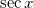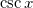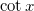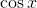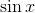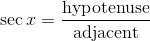, as it is the inverse of thefunction.  This is therefore the answer.